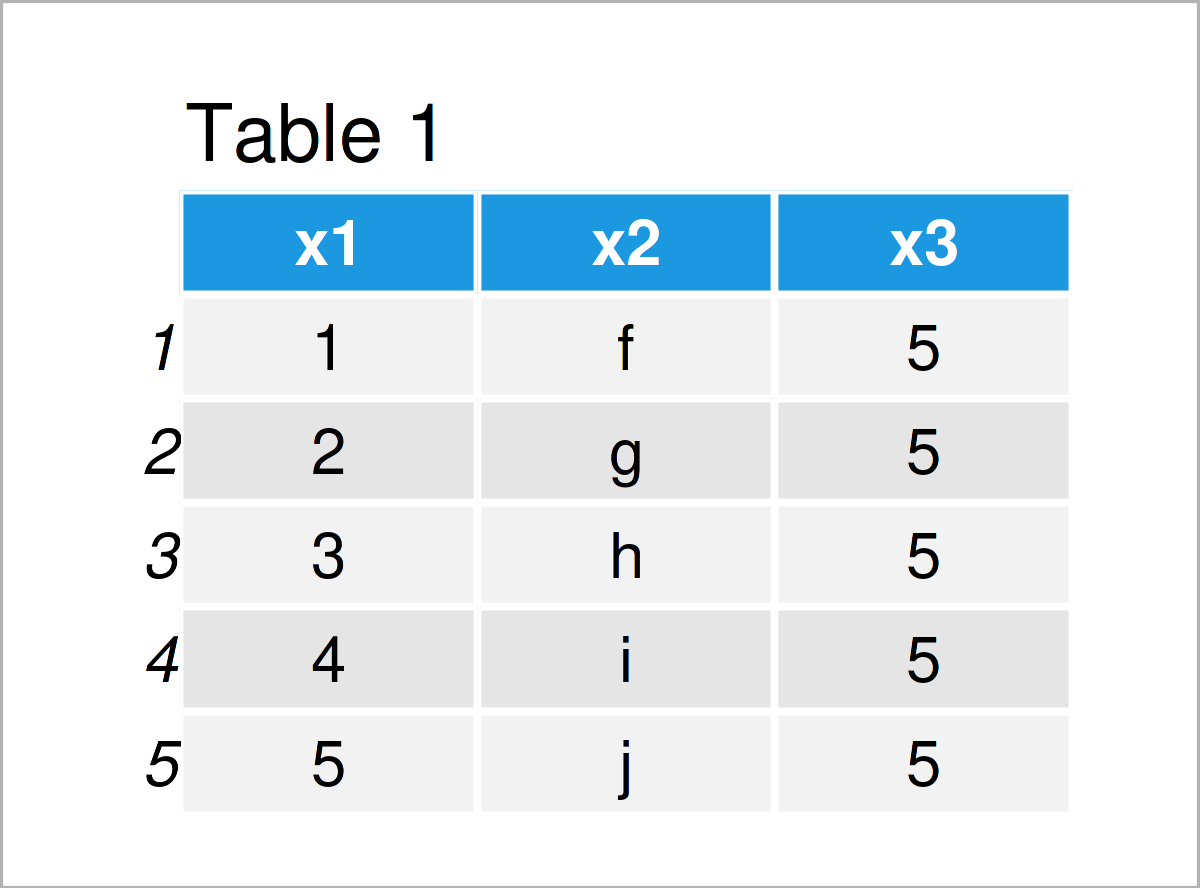# Add Variable Labels to Data Frame in R (2 Examples)

In this article you’ll learn how to assign variable labels to a data frame in the R programming language.

The post will contain this information:

Let’s just jump right in…

## Creation of Example Data

We’ll use the following data frame as a basis for this R programming tutorial:

```data <- data.frame(x1 = 1:5,                # Create example data frame
x2 = letters[6:10],
x3 = 5)
data                                        # Print example data frame```Table 1 shows the structure of our example data frame – It consists of five rows and three columns.

Next, we have to create a named vector that contains the labels for each of the variables in our data frame:

```my_labels <- c(x1 = "My 1st variable contains integers.",  # Create labels
x2 = "My 2nd variable contains characters.",
x3 = "My 3rd variable contains only one value.")
my_labels                                   # Print labels
#                                         x1                                         x2
#       "My 1st variable contains integers."     "My 2nd variable contains characters."
#                                         x3
# "My 3rd variable contains only one value."```

## Example 1: Assign Labels to Data Frame Variables Using Hmisc Package

This example demonstrates how to add text labels to the variables of a data frame object using the Hmisc package.

To be able to use the functions of the Hmisc package, we first need to install and load Hmisc:

```install.packages("Hmisc")                   # Install & load Hmisc
library("Hmisc")```

Furthermore, let’s create a duplicate of our example data frame so that we can keep an original version of our data:

`data1 <- data                               # Duplicate data frame`

Next, we can use the label function of the Hmisc package to print the current labels of our data frame columns:

```label(data1)                                # Check labels of data frame variables
# x1 x2 x3
# "" "" ""```

As you can see, at this point no labels have been assigned. Let’s change that!

The R syntax below uses the as.list, match, and names functions to assign our previously specified named vector as new labels to the variables of our data frame:

```label(data1) <- as.list(my_labels[match(names(data1), # Assign labels to data frame variables
names(my_labels))])```

Let’s use the label function once again to print the updated variable labels:

```label(data1)                                # Check updated labels of data frame variables
#                                         x1                                         x2
#       "My 1st variable contains integers."     "My 2nd variable contains characters."
#                                         x3
# "My 3rd variable contains only one value."```

As you can see, we have added the labels to our data frame.

## Example 2: Assign Labels to Data Frame Variables Using labelled Package

Alternatively to the Hmisc package, we can also use the labelled package.

We first need to install and load the labelled package:

```install.packages("labelled")                # Install labelled package

Once again, I’m creating a duplicate of our example data:

`data2 <- data                               # Duplicate data frame`

Now, we can apply the set_variable_labels function to change the labels of our data frame columns:

```data2 <- set_variable_labels(data2,         # Assign labels to data frame variables
.labels = my_labels)```

Let’s use the label function of the Hmisc package to print our labels:

```label(data2)                                # Check updated labels of data frame variables
#                                         x1                                         x2
#       "My 1st variable contains integers."     "My 2nd variable contains characters."
#                                         x3
# "My 3rd variable contains only one value."```

The output is exactly the same as in Example 1. However, this time we have used the set_variable_labels function of the labelled package instead of the label function of the Hmisc package.

## Video & Further Resources

Have a look at the following video on my YouTube channel. I show the topics of this tutorial in the video:

Furthermore, you might want to read the related R tutorials that I have published on this website: